# Superpixel Posterization

Today's blog post was inspired by an example written by my friend and Image Processing Toolbox developer, Alex Taylor. A few years back, Alex tinkered with using toolbox algorithms to achieve a pseudo-artistic "posterization" effect, like this:

imshow('eddins-horn-1000.png')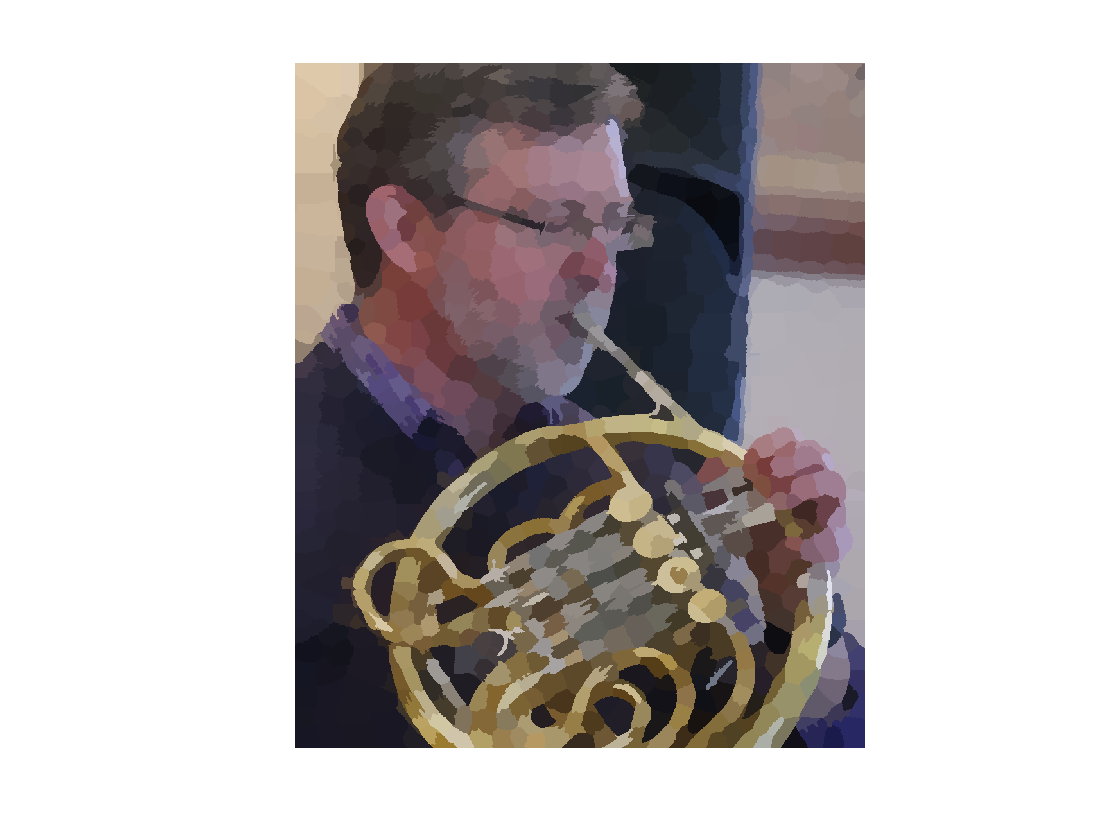I thought the technique was cool and worth showing. I also noticed that the example uses several Image Processing Toolbox functions that haven't been in the product very long, including:

rgb2lab

lab2rgb

imoverlay

superpixels

So I realized this would be a good chance to introduce you to some recent toolbox additions that you might not know about.

### rgb2lab and lab2rgb

The two functions rgb2lab and lab2rgb were introduced in R2014b. They convert between an RGB space and the CIE L*a*b* space. You could perform this conversion previously using the functions makecform and applycform, but it was awkward. These functions support both sRGB and Adobe RGB (1998).

I have mentioned these functions in a couple of previous blog posts, including "Displaying a color gamut surface" and "Out-of-gamut colors."

The function boundarymask, added in R2016a, produces a binary image whose foreground pixels delineate either the boundaries between adjacent labels, if you give it a label matrix, or the boundaries between the foreground and background, if you give it a binary image. Here's an example.

I = imread('rice.png');
subplot(1,2,1)
imshow(I)
title('Original image')
subplot(1,2,2)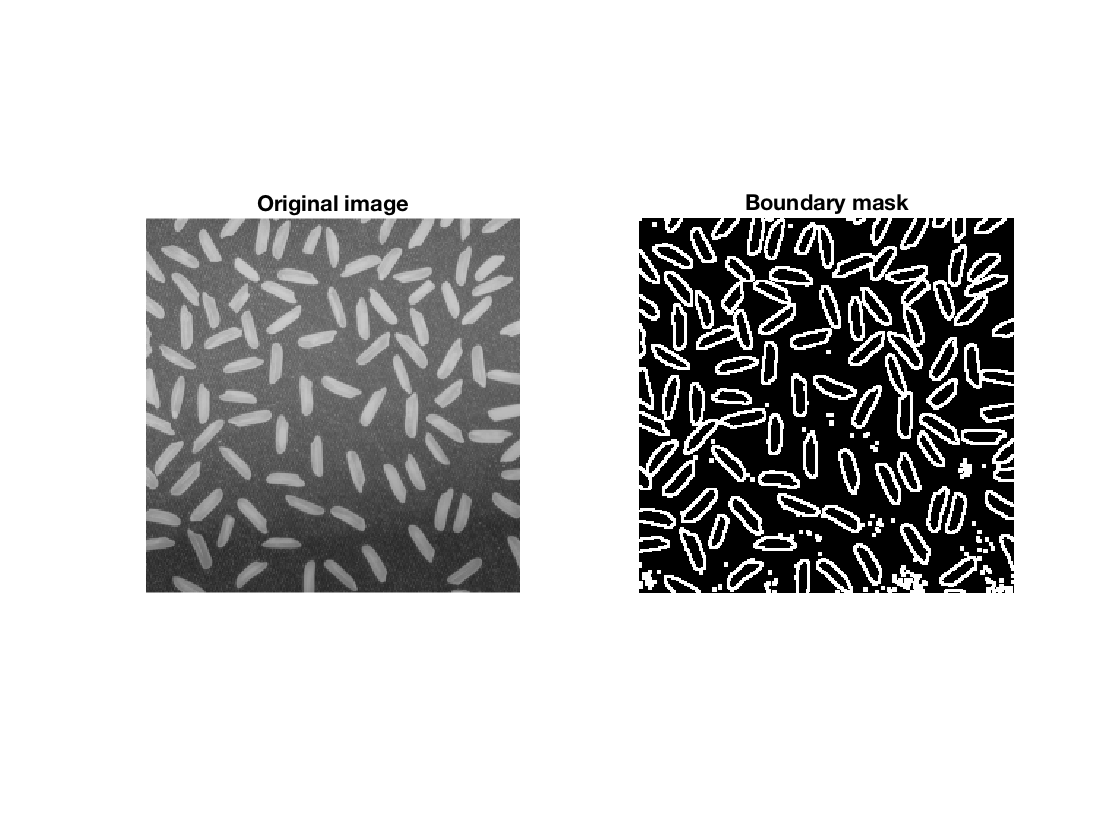### imoverlay

The function imoverlay, added in R2016a, is useful for highlighting a subset of pixel locations in an image. A good example would be to show the boundary mask computed above on top of the original image.

I_overlay = imoverlay(I,mask,'yellow');
imshow(I_overlay)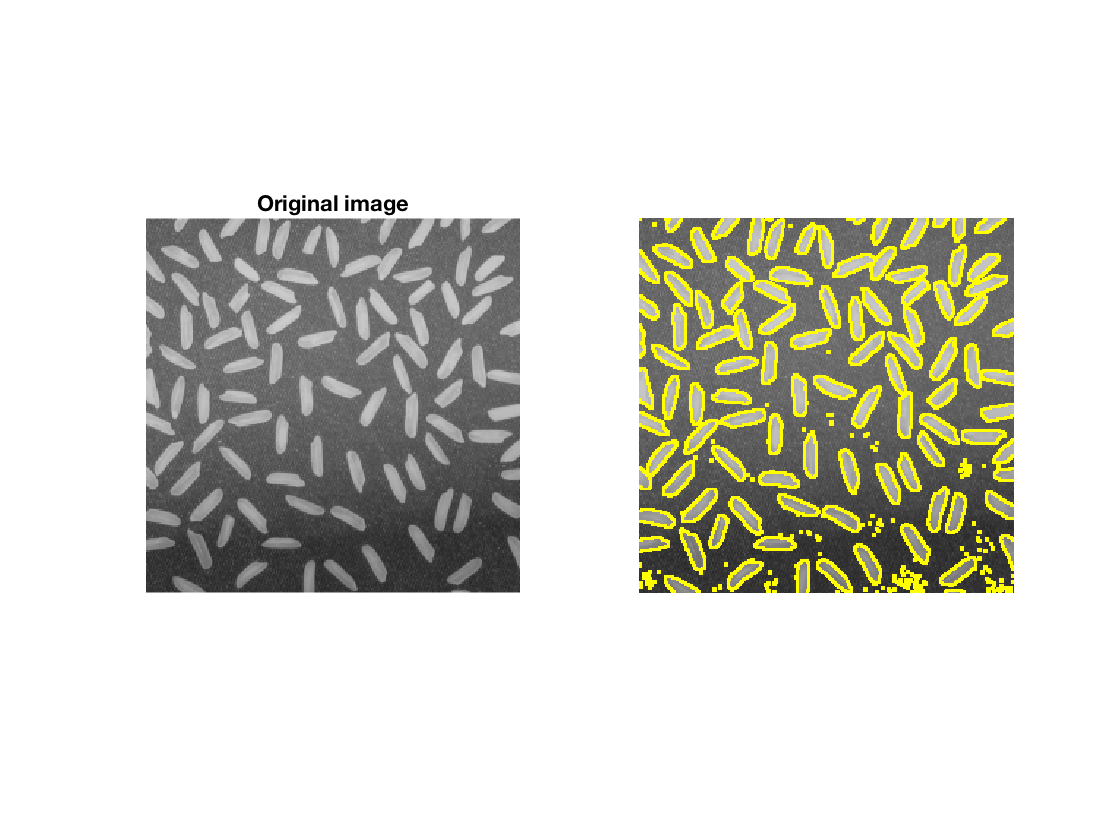In my first year of writing this blog (2006), I wrote my own function with this name and submitted it to the File Exchange. Now that the Image Processing Toolbox has its own version, I'll probably remove mine from the File Exchange soon.

### superpixels

A "superpixel" is simply a group of connected pixels that have similar colors. Computing superpixels has found a regular place in a variety of image analysis and computer vision tasks. The Image Processing Toolbox function superpixels, introduced in R2016a, computes these groups. Here's an example that computes the superpixels and then uses both boundarymask and imoverlay to visualize them.

A = imread('kobi.png');
L = superpixels(A,1500);
clf
imshow(B)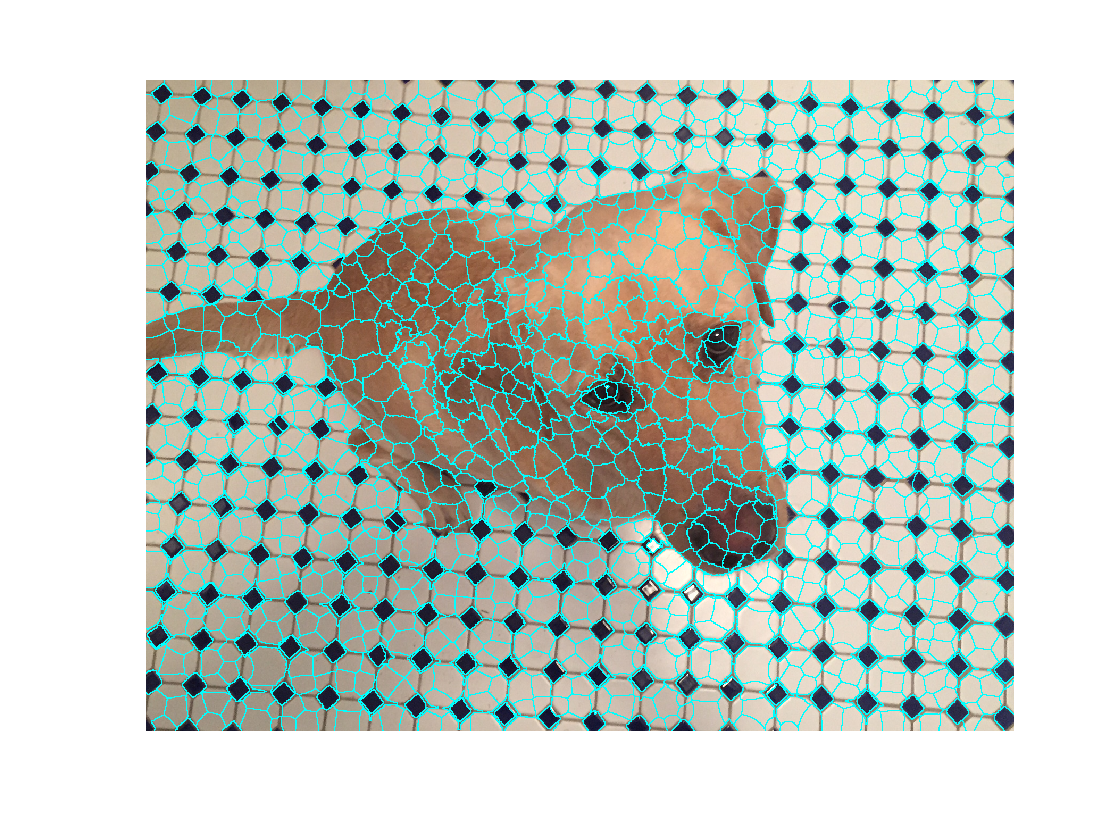### Superpixel Posterization

The superpixel posterization method, as implemented by Alex, starts by using superpixels to compute clusters of like pixels.

Here's how it works.

A = imread('eddins-horn.png');
imshow(A)
title('Original image')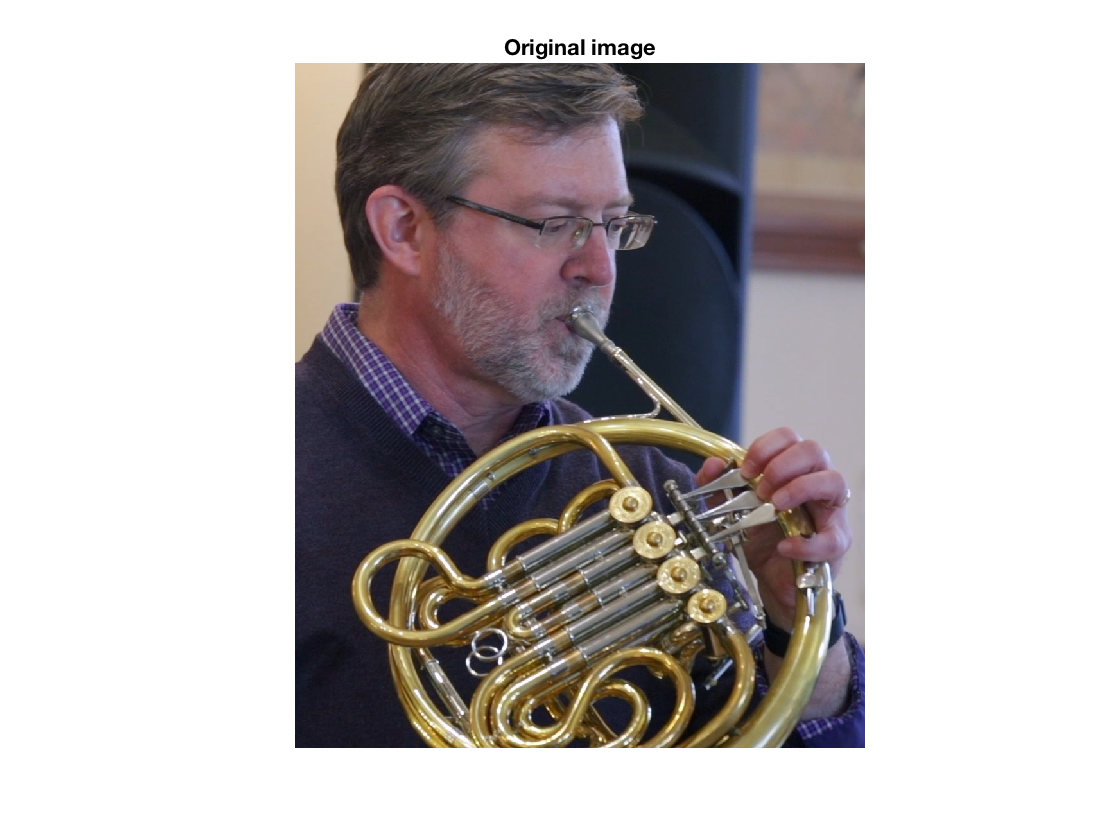Next, compute and visualize the superpixel clusters.

[L,N] = superpixels(A,1000);
imshow(imoverlay(A,BW,'cyan'))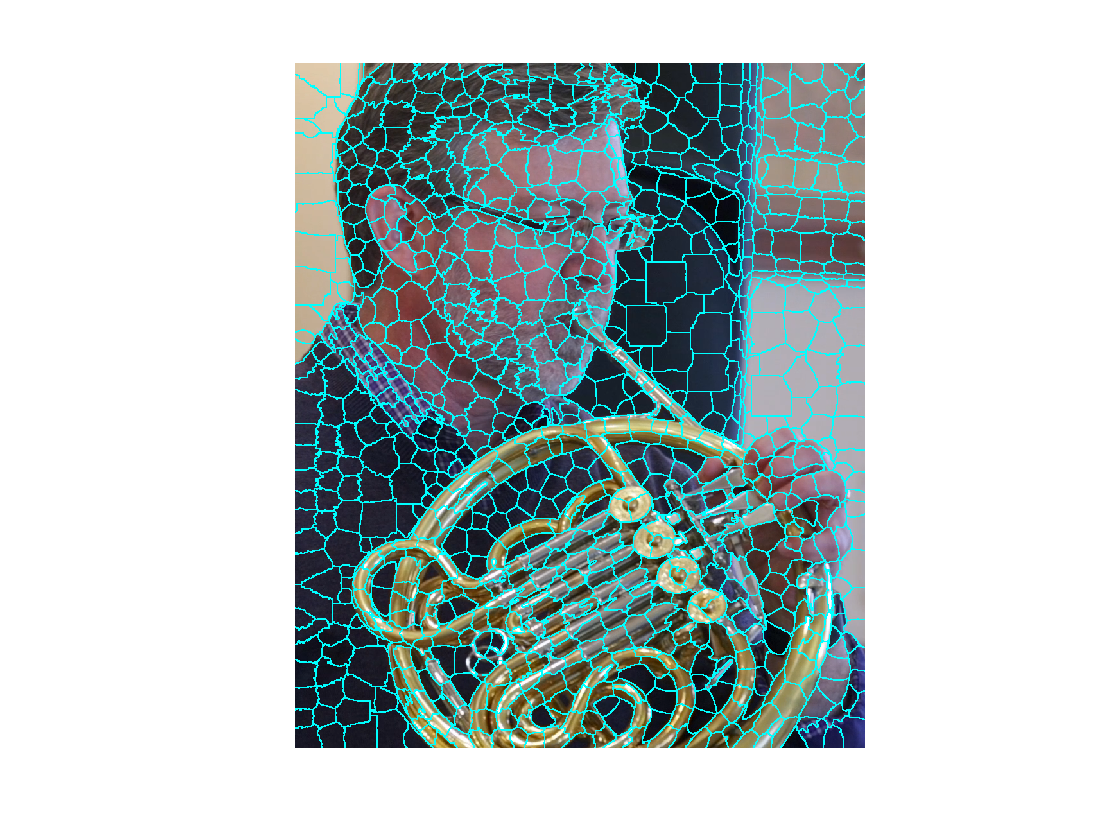In the next step, I want to replace the pixels in each superpixel cluster with the mean of the cluster's colors. But I want to compute the mean in L*a*b* space, so I start by converting from RGB to L*a*b*.

Alab = rgb2lab(A);


I'll use the function label2idx to compute the indices of the pixels in each superpixel cluster. That will let me access the red, green, and blue component values using linear indexing.

pixel_idx = label2idx(L);


For each of the N superpixel clusters, use linear indexing to access the red, green, and blue components, compute the corresponding means, and insert those mean values into the corresponding pixel positions in the L*a*b* output image.

Aplab = Alab;
Ln = numel(L);
for k = 1:N
idx = pixel_idx{k};
Aplab(idx) = mean(Alab(idx));
Aplab(idx+Ln) = mean(Alab(idx+Ln));
Aplab(idx+2*Ln) = mean(Alab(idx+2*Ln));
end


Finally, convert back to RGB space.

Ap = lab2rgb(Aplab);
imshow(Ap)|

### 댓글

댓글을 남기려면 링크 를 클릭하여 MathWorks 계정에 로그인하거나 계정을 새로 만드십시오.